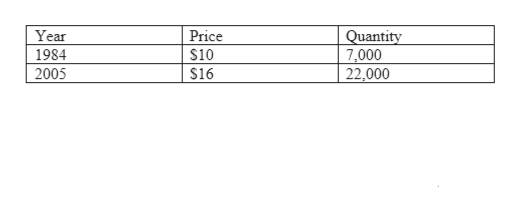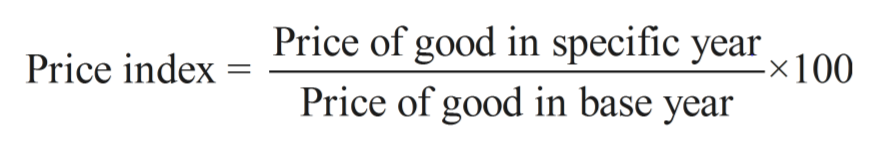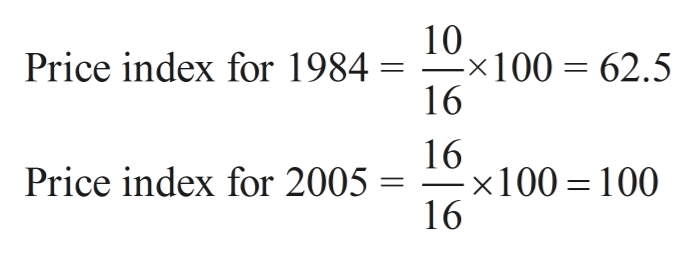Suppose that in 1984 the total output in a single-good economy was 7,000 buckets of chicken. Also suppose that in 1984 each bucket of chicken was priced at \$ 10. Finally, assume that in 2005 the price per bucket of chicken was \$ 16 and that 22,000 buckets were produced. Instructions: In part a enter your answer as an index number rounded to 1 decimal place. In parts b-c, enter your answer as whole numbers. What is the GDP price index for 1984, using 2005 as the base year? By what percentage did the price level, as measured by this index, rise between 1984 and 2005? What were the amounts of real GDP in 1984 and 2005?

Question

Suppose that in 1984 the total output in a single-good economy was 7,000 buckets of chicken. Also suppose that in 1984 each bucket of chicken was priced at \$ 10. Finally, assume that in 2005 the price per bucket of chicken was \$ 16 and that 22,000 buckets were produced. Instructions: In part enter your answer as an index number rounded to 1 decimal place. In parts b-c, enter your answer as whole numbers.

What is the GDP price index for 1984, using 2005 as the base year?

By what percentage did the price level, as measured by this index, rise between 1984 and 2005?

What were the amounts of real GDP in 1984 and 2005?

Step 1

The following information is given:help_outlineImage TranscriptioncloseYear Price Quantity 7,000 22,000 1984 \$10 2005 \$16 fullscreen
Step 2

GDP Price Index can be calculated by the following formula:help_outlineImage TranscriptionclosePrice of good in specific year x100. Price index Price of good in base year fullscreen
Step 3

The GDP Price Index for the years 1984 and 2005 (ta...help_outlineImage Transcriptionclose10 x100 62.5 16 Price index for 1984 = - 16 x100 100 16 Price index for 2005 = fullscreen

Want to see the full answer?

See Solution

Want to see this answer and more?

Our solutions are written by experts, many with advanced degrees, and available 24/7

See Solution
Tagged in